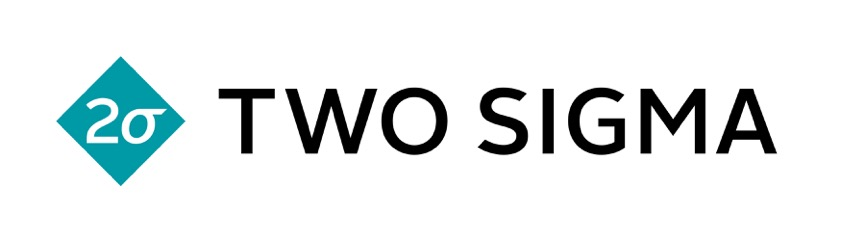# Stephen Tu on Least Squares Policy Iteration

## New York - August 31, 2017

Description

Policy iteration is a classic dynamic programing algorithm for solving a Markov Decision Process (MDP). In policy iteration, the algorithm alternates between two steps: 1) a policy evaluation step which, given the current policy, computes the state-action value function (commonly known as the Q-function) for the policy, and 2) a policy improvement step, which uses the Q-function to greedily improve the current policy. When the number of states and actions of the MDP is finite and small, policy iteration performs well and comes with nice theoretical guarantees. However, when the state and action spaces are large (possibly continuous), policy iteration becomes intractable, and approximate methods for solving MDPs must be used.

Least Squares Policy Iteration (LSPI) is one method for approximately solving an MDP. The key idea here is to approximate the Q-function as a linear functional in a lifted, higher dimensional space, analogous to the idea of feature maps in supervised learning. Plugging this approximation into the Bellman equation gives a tractable linear system of equations to solve for the policy evaluation step. Furthermore, the policy improvement step remains the same as before.

This talk describes LSPI and some of its subtleties. One subtlety arises due to the fact that the Bellman operator is not necessarily invariant on our approximate function class, and hence an extra projection step is typically used to minimize the Bellman residual after projecting back on the function space. Furthermore, in order to build intuition for LSPI, I will also talk about what the LSPI algorithm does in the context of a well studied continuous optimal control problem known as the Linear Quadratic Regulator (LQR).

Bio

Stephen Tu is a PhD student in the EECS department at UC Berkeley, studying problems in the intersection of optimization, control theory, and statistics. This summer, he is interning at the Google Brain team in NYC, which has sparked his interest in reinforcement learning.

Audio

SlidesThe New York Chapter would like to thank TwoSigma for helping to make this meetup possible.